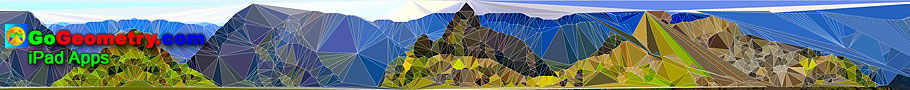iPad Apps for Geometry, College, High School, K-12, Educators: Table of ContentDynamic Geometry 1481. Five Tangential or Circumscribed Quadrilaterals, Pitot Theorem, Congruence, Step-by-step Illustration. GeoGebra, iPad. Dynamic Geometry 1480. Japanese Theorem for Cyclic Polygon, Sangaku, Triangulation, Non-intersecting Diagonals, Sum of Inradii, Invariant, Step-by-step Illustration. GeoGebra, iPad. Dynamic Geometry 1479. Triangle, Circumcircle, Angle Bisector, Perpendicular Bisector, Chord, Concyclic Points, Parallel Lines,, Step-by-step Illustration. GeoGebra, iPad. Dynamic Geometry 1478. Cyclocevian, Reuschle-Terquem Theorem, Concurrent Cevians, Triangle, Circumcircle, Secant line, Step-by-step Illustration. GeoGebra, iPad. Dynamic Geometry 1477. Miquel's Pentagram Theorem, Pentagon, Triangle, Circumcircles, Concyclic Points, Step-by-step Illustration. GeoGebra, iPad. Dynamic Geometry 1476. Droz-Farny Line Theorem, Triangle, Orthocenter, Perpendicular, Collinear Midpoints, Step-by-step Illustration. GeoGebra, iPad. Dynamic Geometry 1475. Clifford Intersecting Circles Theorem, Step-by-step Illustration, GeoGebra, iPad. Dynamic Geometry 1474. Butterfly Theorem, Circle, Chords, Midpoints, Step-by-step Illustration. Dynamic Geometry 1473. Kosnita's Theorem, Triangle, Four Circumcenters, Concurrent Line, Step-by-step Illustration. Dynamic Geometry 1472. Cyclic Quadrilateral, Perpendicular Diagonals, Rectangle, Step-by-step Illustration. Dynamic Geometry 1471. Equilateral Triangle, Inside/Outside Point, Incenters, Tangency Points, Concurrent Lines, Step-by-step Illustration. Dynamic Geometry 1470. Tangential Quadrilateral, Incircles, Tangent, Parallel, Rhombus, Step-by-step Illustration. Dynamic Geometry 1469. Triangle, Circumradius, Inradius, Midpoints, Arcs, Sum of Distances, Step-by-step Illustration. Dynamic Geometry 1468. Steiner's Theorem, Triangle, Circumradius, Inradius, Sum of Exradii, Step-by-step Illustration. Geometry Problem 1467. Square, Rectangle, Triangle, Area. Geometry Problem 1466. Tangential Quadrilateral, Newton Line, Incenter, Midpoint, Diagonal, Collinear Points. Step-by-step Illustration using GeoGebra. Geometry Problem 1465. Tangential Quadrilateral, Incenter, Inscribed Circle, Equal Sum of Areas. Step-by-step Illustration using GeoGebra. Geometry Problem 1464. Quadrilateral, Interior Point, Midpoint of Sides, Equal Sum of Areas. Step-by-step Illustration using GeoGebra. Geometry Problem 1463. Parallelogram, Interior Point, Opposite Triangles with Equal Sum of Areas. Step-by-step Illustration using GeoGebra. Geometry Problem 1462. Newton-Line, Newton-Gauss Line, Complete Quadrilateral, Midpoints of Sides and Diagonals, Collinear Points. Geometry Problem 1461. Quadrilateral, Triangle, Angles, 30-60 Degree, Congruence, Auxiliary Lines. Dynamic Geometry 1460. Newton-Gauss Line, Complete Quadrilateral, Midpoints of Diagonals, Collinear Points, Step-by-step Illustration using GeoGebra. Geometry Problem 1459. Two Triangles, Orthocenter, Midpoint, Perpendicular, Step-by-step Illustration using GeoGebra. Geometry Problem 1458. Triangle, Incircles, Excircle, Area, Step-by-step Illustration using GeoGebra. Geometry Problem 1457. Altitudes, Circles, Similarity, Product of the Inradii Lengths, iPad. Step-by-step illustration using GeoGebra. Geometry Problem 1456. Altitudes, Orthic Triangle, Circumcircle, Parallel lines, Similarity, Area, iPad. Step-by-step illustration using GeoGebra. Geometry Problem 1455. Nagel Point, Excircles, Incircle, Congruent Segments, iPad. Step-by-step illustration using GeoGebra. Geometry Problem 1454. Intersecting Circles, Perpendicular Lines, Cyclic Quadrilateral, Collinear Points, iPad. Step-by-step illustration using GeoGebra. Dynamic Geometry 1452. Japanese Theorem, Sangaku, Cyclic Quadrilateral, Incenter, Rectangle, Inradius. Step-by-step illustration using GeoGebra. Dynamic Geometry 1451. Orthopole of a Line. Step-by-step illustration using GeoGebra. Dynamic Geometry 1450. Ortholine, Steiner Line, Complete Quadrilateral, Collineal Orthocenters. Step-by-step illustration using GeoGebra. Dynamic Geometry 1449. Salmon Line. Step-by-step illustration using GeoGebra. Dynamic Geometry 1448. Simson Line. Step-by-step illustration using GeoGebra. Dynamic Geometry Problem 1447. Outer Vecten Point. Step-by-step illustration using GeoGebra. Art of Problem 1438. Circular Dissolve, Space Fractal, iPad, Mobile Apps. Dynamic Geometry Problem 1446. Lemoine Line, triangle, circumcircle, tangent, collinear points. Step-by-step animation using GeoGebra. Geometry Problem 1432. Tangent Circles, Secant, Tangent Lines, Proportionality, Similarity, Poster Typography, iPad. Art of Problem 1143. Triangle, Circle, Tangent, Reflection, Collinearity, iPad Apps, Mobile Apps. Art of Geometry Problem 1404.Three Intersecting Circles and Circle Patterns using Mobile Apps. Dynamic Geometry Problem 1445. Van Aubel's theorem, Quadrilateral and Four Squares, Centers. Step-by-step animation using GeoGebra. Geometry Problem 1433. Tangent Circles, Diameter, Perpendicular, Midpoint, Measurement, Poster Typography, iPad Apps. Art of Problem 1384. Triangle, Orthocenter, Circle, Angle Bisector, Perpendicular, iPad Apps, Mobile. Etching of the Taj Mahal. Using iPad Apps. Art and typography of Geometry Problem 1099. Using iPad Apps. Typography of Geometry Problem 970: Equilateral Triangle, Circle using iPad Apps. Art of Geometry Problem 1038. Using iPad Apps. Geometric Art of Equal Incircles theorem using iPad Apps. Go to Page: Previous | 1 | 2 | 3 | 4 | 5 | 6 | 7 | 8 | 9 | 10 | 11 | 12 | 13 | 14 | 15 | 16 | 17 | 18 | 19 | 20 | Next

Home | Sitemap | Software | Email
Last updated: May 24, 2020